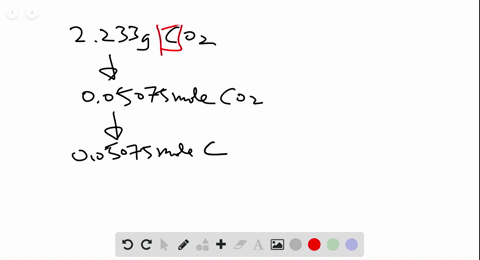🎉 The Study-to-Win Winning Ticket number has been announced! Go to your Tickets dashboard to see if you won! 🎉View Winning Ticket### Ferrocene, synthesized in 1951 , was the first or…

06:49Problem 123

# What mass percents of ammonium sulfate, ammonium hydrogen phosphate, and potassium chloride would you use to prepare 10$/ 10 / 10$ plant fertilizer (see Problem 3.122$) ?$

## Discussion

You must be signed in to discuss.

## Video Transcript

some for this problem were given a long chemical equation involving ammonium sulfate and ammonia. And you want to find how many kilograms of ammonia are needed to produce some amount of ammonium sulphate. So the first step in solving this problem is we're gonna have to find them warm masses of both ammonia and ammonium sulfate, so we can then use them in our dimension analysis. Later on, let's first do ammonium sulfate, which looks like this. So we have to night regions his fortune, but no one grand from all we have eight hydrogen, which is one point alo eight grams we have a cell for which is 32.7 grams per mole. And then finally we have four oxygen's, which are 16.0 grams promote. So when he wants by all this out, we'll get that. Our answer is 132 0.14 grams per mole, so that gives us a molar mass of the first compound. And next we need to do pneumonia. Well, ammonia is just one nitrogen and then three hydrants. When you multiply that out, we'll get 17 03 grams. So now that we have the more masses of both substances. We can then do some basic dimensional analysis to go from kilograms of ammonium sulfate, two kilograms of ammonia. So our first unit is one point over old times 10 to the five kilograms ammonium. So feet we first need to convert station two grams, you know, in one kilogram the 2000 grams. And from there we want to convert this into moles of ammonium sulfate. And we can do this by dividing by the molar mass as we calculated to be 132.4 grams for all. And now we want to find the multiple ratio of ammonia to ammonia ammonium sulphate, so that we can get moles of ammonia. Well, if you look at the chemical equation, two moles of ammonia are required to produce one more of ammonium selfie. So the multiple ratios to 20 right, that the last few steps we need to then divide, multiply by the molar mass of ammonia. So we get grams of ammonia and calculated at the more massive 17.3 grams from, and then finally divide by 1000. So we get the final answer in kilograms and when you multiply all of this out, you get that. The final answer. 2.58 games 10 to before kilograms of ammonia. That is the final answer.

#### You're viewing a similar answer. To request the exact answer, fill out the form below:

Our educator team will work on creating an answer for you in the next 6 hours.